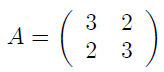## Pages

Python Numpy scipy , matplotlib... Exercise - High School Grade 11

#### Exercise 550|| Solution

Given a square matrix A and a vector V:

Give the Python code numpy which calculates the product AV (the matrix product and not the term to term product).

#### Exercise 551 || Solution

Given two matrixes A and B:Give the numpy python code which calculates t(B)A (the inner product or dot product)

#### Exercise 552 || Solution

For Given Matrix A:1.  Give the python numpy code that compute the reverse B of matrix A
2. To ensure the result, calculate B.dot (A)

#### Exercise 553 || Solution

Give the Python code matplotlib which draws the line D (A, B) passing through the two points A (1,2) and B (2,3).

#### Exercise 554 || Solution

Give the python code numpy which generates the matrix: A = np.array ([0, 1, 2, 3, 4, 5, 6, 7, 8, 9]) via the linespace() method.

#### Exercise 555 || Solution

Give the python code numpy which transforms the matrix: A = np.array ([1, 2, 3, 4, 5, 6, 7, 8, 9]) into matrix : B = np.array([[1, 2, 3], [4, 5, 6],[7, 8, 9]])

#### Exercise 556 || Solution

By using the arange() method, giv a numpy python code to get the matrix: A = np.array([ 1 3 5 7 9 11 13]).

#### Exercise 557 Solution

Consider the following matrix: A = np.array ([[3, -1], [-1, 4], [7,11]]) Give the Python code numpy which displays the rows of the matrix as follows:

line number 1 is: [3 -1]

line number 2 is: [-1 4]

line number 3 is: [7 11]

#### Exercise 558 || Solution

give the python code numpy which changes the value 11 to 22 in the matrix: A = = np.array ([[5, 2], [3, 11], [8.4]])

## Python Exercises With Solutions

1. Python Arithmetic Exercises
2. Equations & System Of Equations
3. Polynomials and Functions
4. Python Arithmetic Exercises
5. Numpy, Matplolib, Sympy , Scipy - Exercises
6. Python Arithmetic Exercises

Younes Derfoufi

## Sample Text